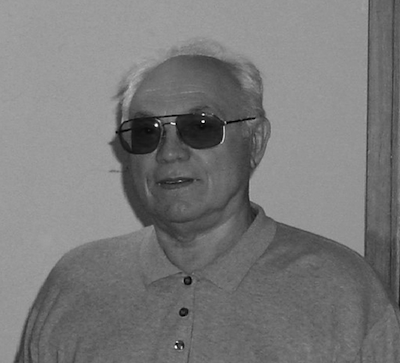The world’s mathematical community lost one of its most prominent members when Professor Anatolii Vladimirovich Skorokhod died on January 3, 2011. Anatolii Skorokhod was born on September 10, 1930 in Nikopol, Ukraine. In 1948, he graduated from secondary school (with a gold medal) and entered the Kyiv State University, where he specialized in probability theory at the Department of Mathematical Analysis, under the considerable influence of Professors B.V. Gnedenko and I.I. Gikhman. In one of his first papers (1954) Skorokhod clarified the structure of densities of stable distributions.

In the early 1950s, the atmosphere at Kyiv University was far from friendly, which resulted finally in B.V. Gnedenko’s departure. Foreseeing this, Gnedenko wrote to A.N. Kolmogorov: “Dear Andrei, one more matter. Here we have a graduating student, very able in my view, Skorokhod Anatolii Vladimirovich, 22 years of age. Things are going in such a way that it would be better for him to leave and attend postgraduate courses elsewhere. If there is a possibility, by the ministry, to assign him to Moscow University for postgraduate study, then you will do a good deed both for the student and for science…” (From a letter of B.V. Gnedenko to A.N. Kolmogorov, October 30, 1952.)

Thus, by the efforts of Kolmogorov, Moscow State University acquired three post-graduate students: A. V. Skorokhod (supervisor E.B. Dynkin), V.S. Korolyuk and V.S. Mikhalevich. All of them would later become Members of the Academy of Sciences of Ukraine.

A.V. Skorokhod wrote his Candidate dissertation, Limit theorems for random processes, at Moscow State University, in 1956, and returned to the Kyiv State University. In 1962 he completed his Doctoral dissertation, SDE and limit theorems for stochastic processes, and was made Professor of the Kyiv State University the following year. A year later he moved to the Institute of Mathematics of Academy of Sciences of Ukraine as Head of Department of the theory of stochastic processes. He became a Corresponding member of the Ukrainian Academy of Sciences in 1967 (and an Academician in 1985), and was awarded the Ukrainian State Prize in Science and Technology in 1982 and 2003. In 1985 Skorokhod moved to Michigan State University, USA, and in 2000 he was elected to the American Academy of Arts and Sciences.

A.V. Skorokhod’s contributions to probability theory, mathematical statistics, and the theory of stochastic processes, were fundamental, in particular in the areas: limit theorems for stochastic processes; stochastic differential equations; probability distributions in infinite dimensional spaces; stochastic integration of anticipating functions; and asymptotic behavior of dynamic systems under random perturbations.

In the 1950s A.V. Skorokhod began a large cycle of works on limit theorems for stochastic processes. He proposed an original method of studying limit theorems, based on two profound ideas: the method of a single probability space and introduction of several topologies on the space of functions without discontinuities of the second kind. His main idea in the area of limit theorems was to introduce a topology which would make this space a Polish space (complete separable metric space). Along this line A.V. Skorokhod introduced four topologies; established necessary and sufficient conditions for convergence in those topologies; described compact sets for each topology; and listed the most important examples of continuous functionals.

The most well-known topology is the J1-topology (we call it the Skorokhod topology), which proved to be very useful in the case of typical path spaces.

A.V. Skorokhod obtained many significant results in the theory of stochastic differential equations, namely: the theorem of comparison for linear diffusion, which allowed him to get the first results on the existence of strong solutions for the case of non-Lipschitz drift coefficients; the theory of diffusion processes with boundary conditions of different types; asymptotic behavior of solutions; fundamental results on ergodicity and stability of solutions. We also mention his results on the problem of differentiability of probability measures; the structure of the Radon-Nikodým derivatives for quasi-invariant measures on a Hilbert space.

Along with a great number of scientific papers (about 300), A.V. Skorokhod wrote many books, textbooks, and monographs (more than 25), which are well-known in the probability world and are constantly used both by students and experts in probability theory and the theory of stochastic processes. His last book, with Habib Salehi and Frank Hoppensteadt, was Random perturbation methods with applications in science and engineering (Springer, 2002, Ser. Appl. Math. Sci., vol. 150).

Written by Alex Novikov (University of Technology, Sydney, Australia) and Albert Shiryaev (Steklov Mathematical Institute, Moscow)